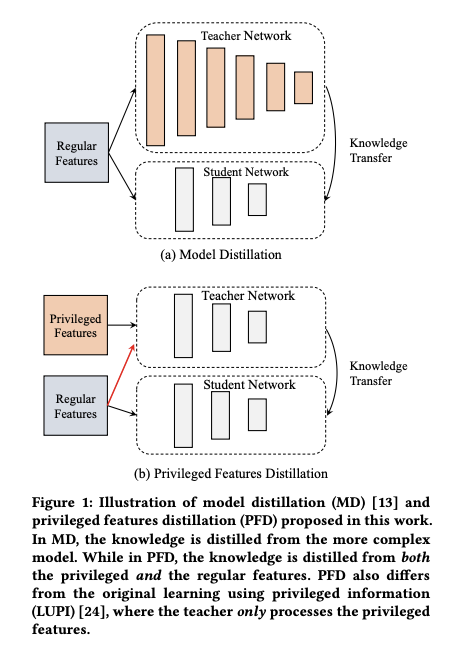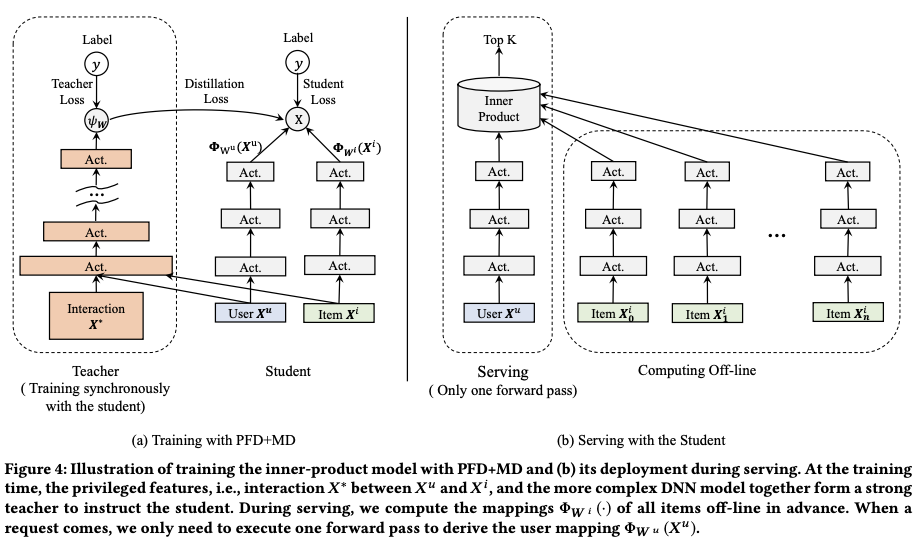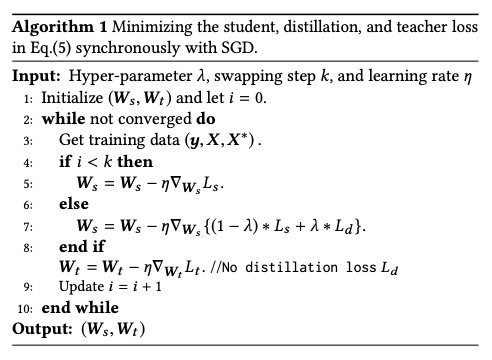## 基本原理

Distillation 可分为 Model Distillation 和 Feature Distillation，其思想都是在训练时同时训练两个模型：teacher 模型和 student 模型，而在 serving 时只用 student 模型。这里的假设是：teacher 模型比起 student 模型，在模型结构上更复杂(Model Distillation) ，或在特征集上更为丰富(Feature Distillation) ；因此其准确率也会比 student 模型要好。$$\min_{W_s} (1-\lambda)L_s(y, f_s(X;W_s))+\lambda*L_d(f_t(X;W_t),f_s(X;W_s)) \tag{1}$$

• $f_s(X; W_s)$ ：student 模型的预估值
• $f_t(X;W_t)$ ： teacher 模型的预估值
• $L_s$ ：student 模型原始的 loss
• $L_d$ ：利用 teacher 模型预估值输出作为 soft label 计算的 distillation loss;
• $\lambda$：平衡 $L_s$ 和 $L_d$ 的超参

$$\min_{W_s} (1-\lambda)L_s(y, f_s(X;W_s))+\lambda*L_d(f_t(X^*;W_t),f_s(X;W_s)) \tag{2}$$

$$\min_{W_s, W_t} (1-\lambda)L_s(y, f_s(X;W_s)) + \lambda*L_d(f_t(X^*;W_t),f_s(X;W_s)) + L_t(y, f_t(X^*;W_t))\tag{3}$$## 训练注意事项

（1）先训练 teacher 网络，再训练 student 网络，也被称为 asynchronous training
（2）同时训练 teacher 网络和 student 网络，也被称为 synchronous training## 实现

tensorflow 提供的一个distillation 的实现 distillation.py，使用见 stack-overflow 上的 这个回答